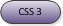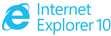# MSCSSMatrix object

Represents a 4×4 homogeneous matrix that enables Document Object Model (DOM) scripting access to Cascading Style Sheets (CSS) 2-D and 3-D Transforms functionality.## DOM Information

### Inheritance Hierarchy

The MSCSSMatrix does not inherit from any class or interface.

## Members

The MSCSSMatrix object has these types of members:

• Methods
• Properties

### Methods

The MSCSSMatrix object has these methods.

Method Description
inverse

Returns a new matrix that is the inverse of the current matrix.

multiply

Returns a new matrix that is the result of current matrix multiplied by the input matrix.

multiplyLeft

Returns a new matrix that is the result of input matrix multiplied by the current matrix.

rotate

Returns a new matrix that is the result of the current matrix multiplied by the rotation matrix corresponding to the input parameters.

rotateAxisAngle

Returns a new matrix that is the result of the current matrix multiplied by the rotation matrix with the given axis and angle.

scale

Returns a new matrix that is the result of the current matrix multiplied by the scale matrix that corresponds to the input parameters.

setMatrixValue

Replaces an existing matrix with the computed matrix that corresponds to the input.

skew

Returns a new matrix that is the result of the current matrix multiplied by the skew matrix that corresponds to the input parameters.

skewX

Specifies a 2-D skew transformation along the x-axis by the given angle.

skewY

Specifies a 2-D skew transformation along the y-axis by the given angle.

toString

Returns a string that corresponds to the matrix.

translate

Returns a new matrix that is the result of the current matrix multiplied by a translation matrix that contains the input parameters.

### Properties

The MSCSSMatrix object has these properties.

Property Access type Description

a

Gets or sets the same value as the m11 property.

b

Gets or sets the same value as the m21 property.

c

Gets or sets the same value as the m12 property.

d

Gets or sets the same value as the m22 property.

e

Gets or sets the same value as the m13 property.

f

Gets or sets the same value as the m23 property.

m11

Gets or sets the value in the first column of the first row of the 4×4 matrix.

m12

Gets or sets the value in the second column of the first row of the 4×4 matrix.

m13

Gets or sets the value in the third column of the first row of the 4×4 matrix.

m14

Gets or sets the value in the fourth column of the first row of the 4×4 matrix.

m21

Gets or sets the value in the first column of the second row of the 4×4 matrix.

m22

Gets or sets the value in the second column of the second row of the 4×4 matrix.

m23

Gets or sets the value in the third column of the second row of the 4×4 matrix.

m24

Gets or sets the value in the fourth column of the second row of the 4×4 matrix.

m31

Gets or sets the value in the first column of the third row of the 4×4 matrix.

m32

Gets or sets the value in the second column of the third row of the 4×4 matrix.

m33

Gets or sets the value in the third column of the third row of the 4×4 matrix.

m34

Gets or sets the value in the fourth column of the third row of the 4×4 matrix.

m41

Gets or sets the value in the first column of the fourth row of the 4×4 matrix.

m42

Gets or sets the value in the second column of the fourth row of the 4×4 matrix.

m43

Gets or sets the value in the third column of the fourth row of the 4×4 matrix.

m44